学哥量化交易学习 持续学习，提高认知，磨炼技艺，从零基础学编程并跨过量化程序交易的大门。 返回首页

0011 迷人的斐波拉契数列，数学到极致就是美，编程同样如此

```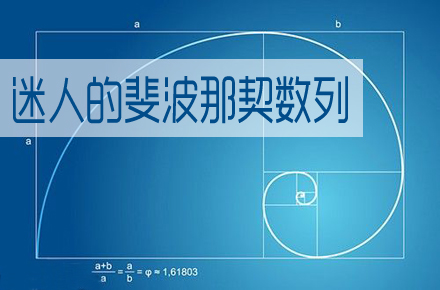a=1
b=1
n=3
while n<=100:
x=a+b
a=b
b=x
n=n+1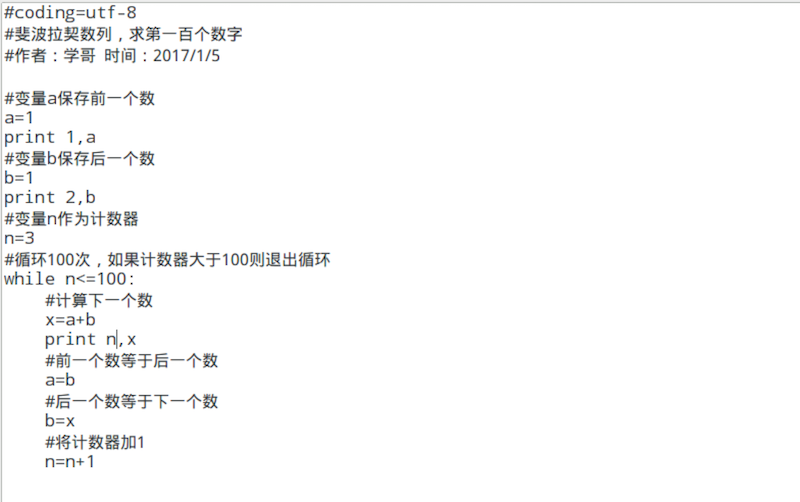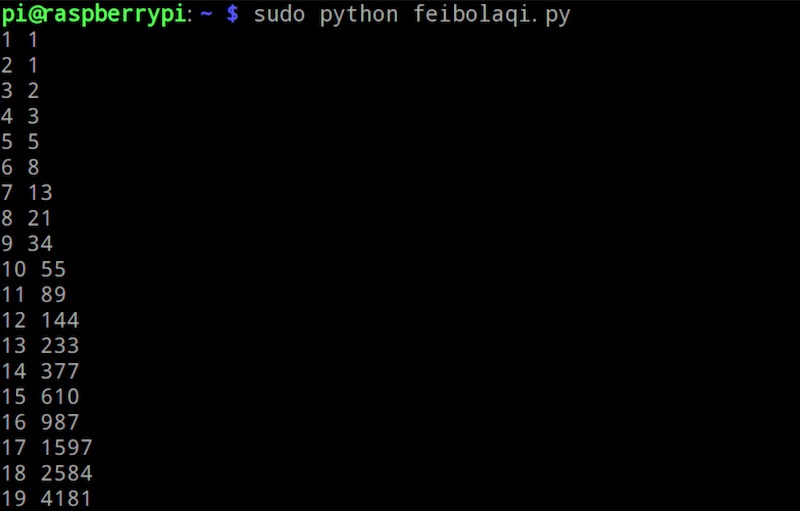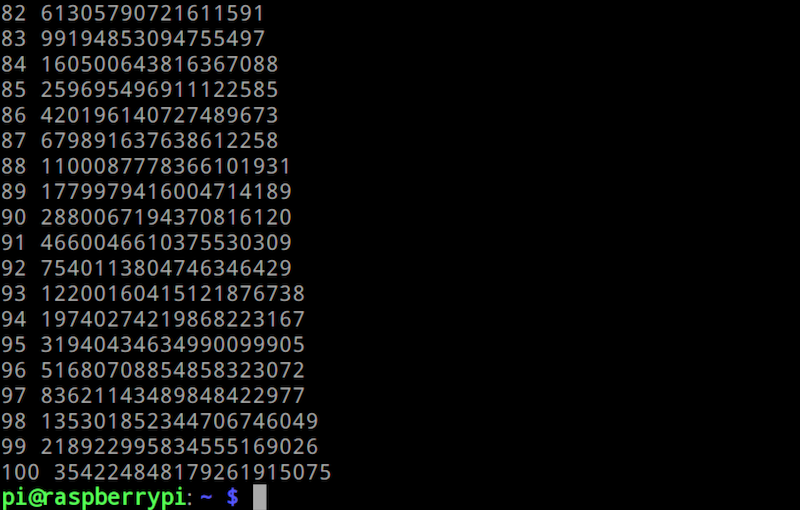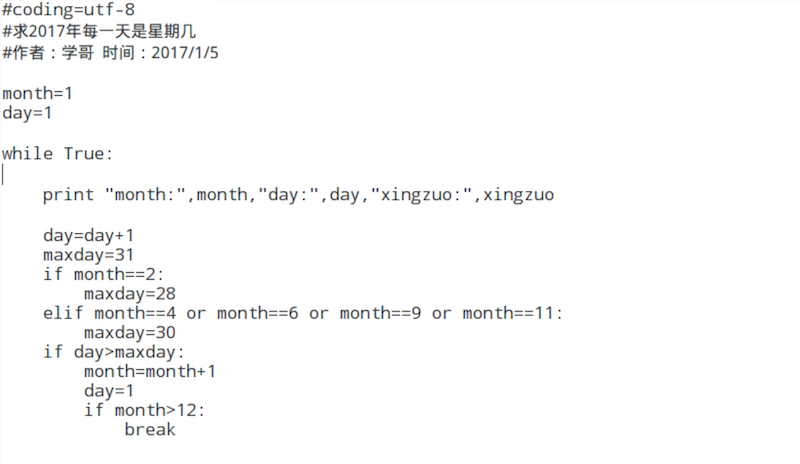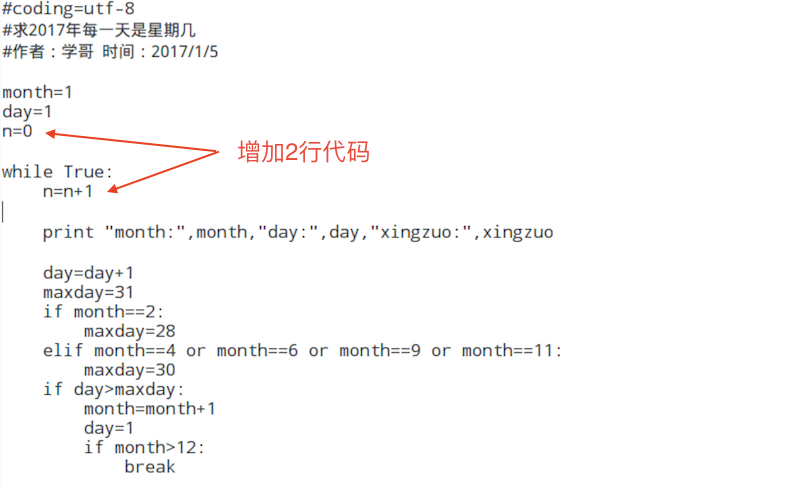week= ( n + 5 ) % 7 + 1
print "2017",month,day,week

week = ( n + x ) % 7 + 1
week = 7
n = 1
7 = ( 1 + x ) % 7 + 1
6 = ( 1 + x ) % 7
6 = 1 + x
5 = x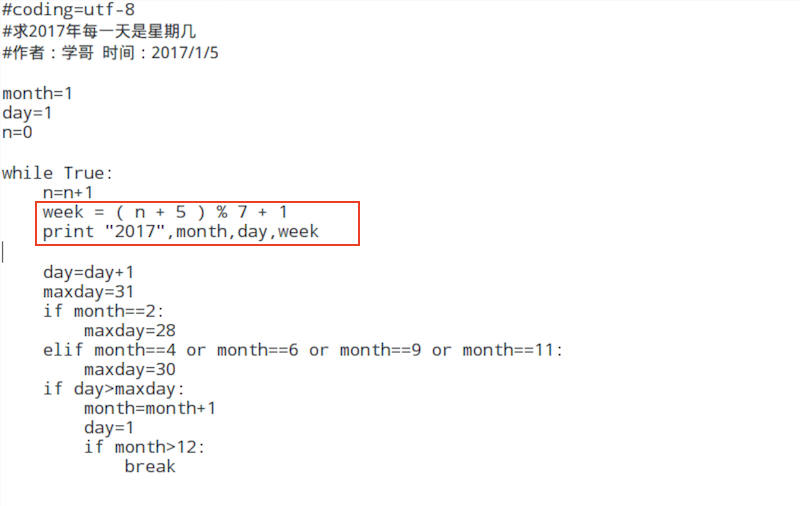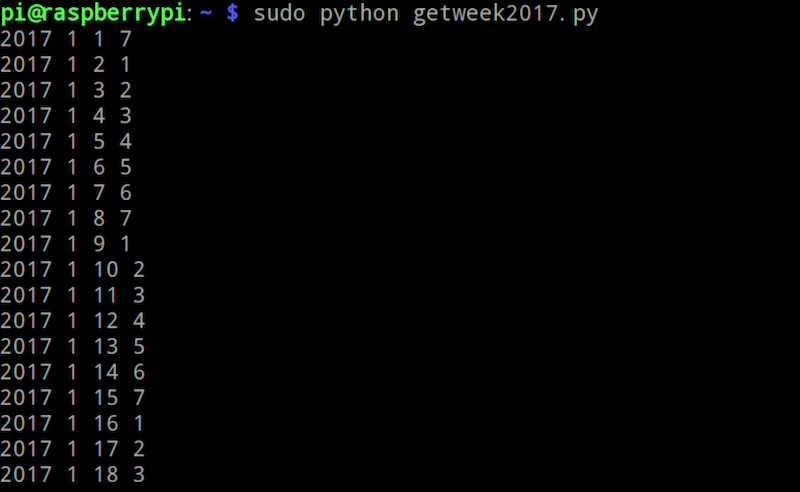week = ( n + x ) % 7 + 1
week = 5
n = 1
5 = ( 1 + x ) % 7 + 1
4 = ( 1 + x ) % 7
4 = 1 + x
3 = x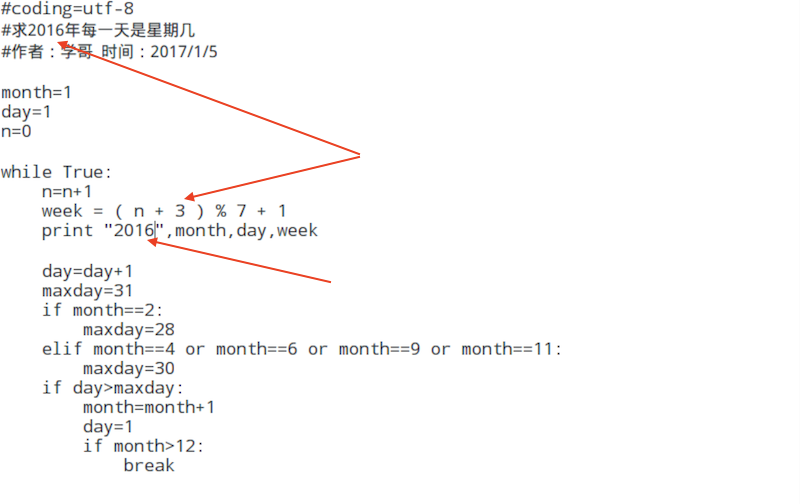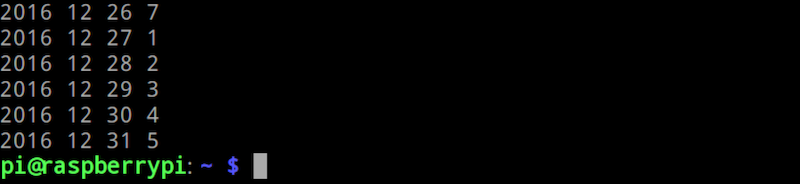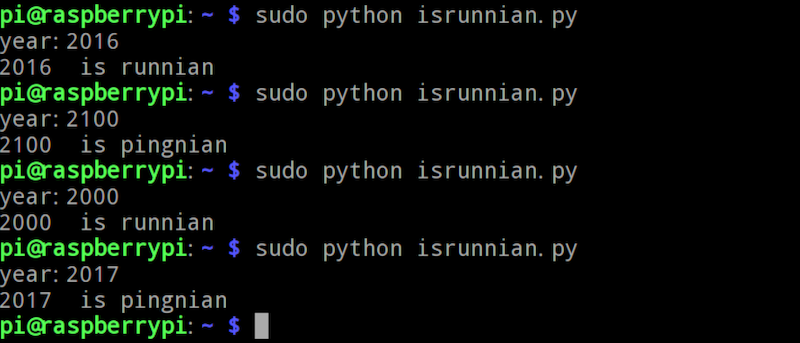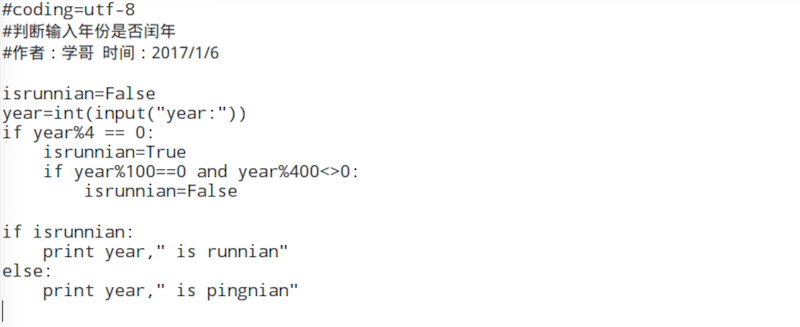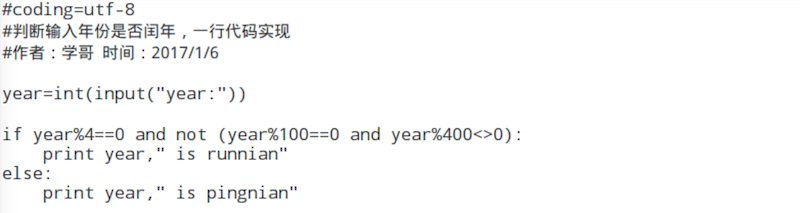```学哥量化交易学习团队 致力于：持续学习，提高认知，磨炼技艺，持续输出高质量的教程和课程，帮助更多的人从零基础学编程并跨过量化程序交易的大门。 零基础学编程Python入门课程 课程包含以下内容： 1.图文教程《零基础学编程入门-从Python到Web网站.pdf》，学哥原创版权，共计1024页高清图文教程。 2.视频课程《零基础学编程Python入门》，学哥原创版权，共计24节课45个高清视频。 3.视频教程《玩转魔方》，学哥原创版权，可以送给孩子，共计16个高清视频。 4.图文教程《如何寻找编程相关的电子书下载资源.pdf》。 5.加入学哥编程学习辅导QQ群在线交流。 客服 10:00-17:00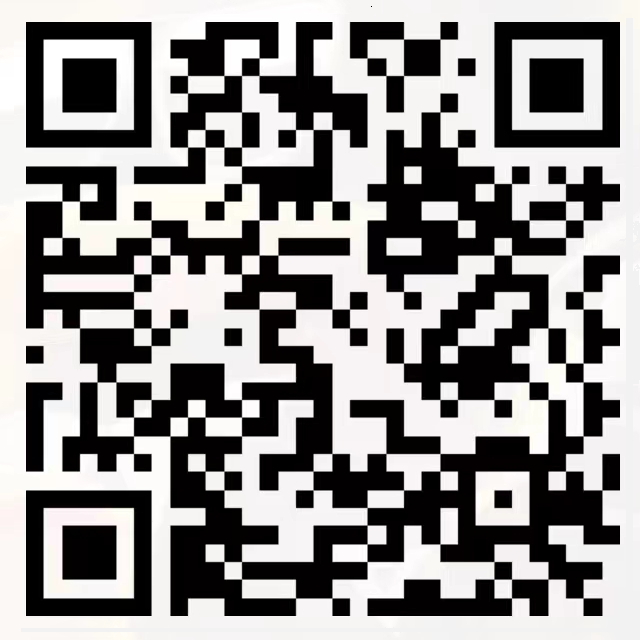QQ: 44556287 微信公众号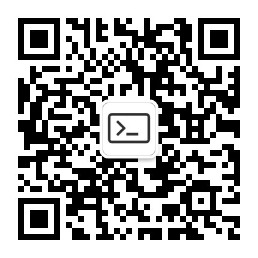学哥量化交易学习

 微信公众号：学哥量化交易学习     QQ群1：603559164    客服QQ号：44556287 工信部备案/许可证编号：沪ICP备15050545号-4